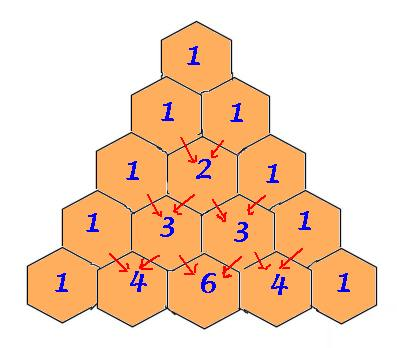# Playing with Numbers worksheet for class 8## myCBSEguide App

CBSE, NCERT, JEE Main, NEET-UG, NDA, Exam Papers, Question Bank, NCERT Solutions, Exemplars, Revision Notes, Free Videos, MCQ Tests & more.

## Playing with Numbers worksheet for class 8 Important Topics

• Introduction
• Numbers in General Form
• Games with Numbers
• Letters for Digits
• Tests of Divisibility## Some important Facts about Playing with Numbers worksheet for class 8

1. Numbers can be written in general form. Thus, a two digit number ab will be written as ab = 10a + b.
2. The general form of numbers are helpful in solving puzzles or number games.
3. The reasons for the divisibility of numbers by 10, 5, 2, 9 or 3 can be given when numbers are written in general form

## NCERT Class 8 Mathematics Solved Worksheets

• Chapter 1: Rational Numbers
• Chapter 2: Linear Equations in One Variable
• Chapter 4: Practical Geometry
• Chapter 5: Data Handling
• Chapter 6: Squares and Square Roots
• Chapter 7: Cubes and Cube Roots
• Chapter 8: Comparing Quantities
• Chapter 9: Algebraic Expressions and Identities
• Chapter 10: Visualising Solid Shapes
• Chapter 11: Mensuration
• Chapter 12: Exponents and Powers
• Chapter 13: Direct and Inverse Proportions
• Chapter 14: Factorisation
• Chapter 15: Introduction to Graphs
• Chapter 16: Playing with Numbers

## Letters for Digits

Here we have puzzles in which letters take the place of digits in an arithmetic ‘sum’, and the problem is to find out which letter represents which digit; so it is like cracking a code. Here we stick to problems of addition and multiplication.

Here are two rules we follow while doing such puzzles.

1. Each letter in the puzzle must stand for just one digit. Each digit must be represented by just one letter.
2. The first digit of a number cannot be zero. Thus, we write the number “sixty three” as 63, and not as 063, or 0063

To download Printable worksheets for class 8 Mathematics and Science; do check myCBSEguide app or website. myCBSEguide provides sample papers with solution, test papers for chapter-wise practice, NCERT solutions, NCERT Exemplar solutions, quick revision notes for ready reference, CBSE guess papers and CBSE important question papers. Sample Paper all are made available through the best app for CBSE students and myCBSEguide website.Test Generator

Create question papers online with solution using our databank of 5,00,000+ questions and download as PDF with your own name & logo in minutes.